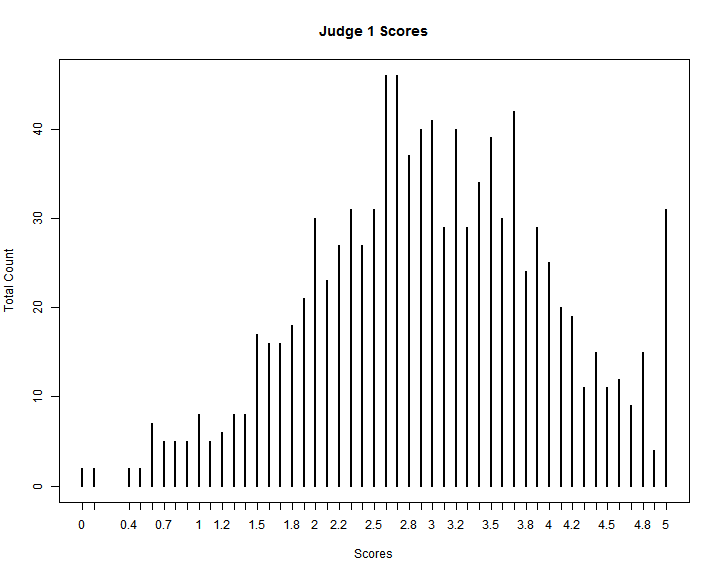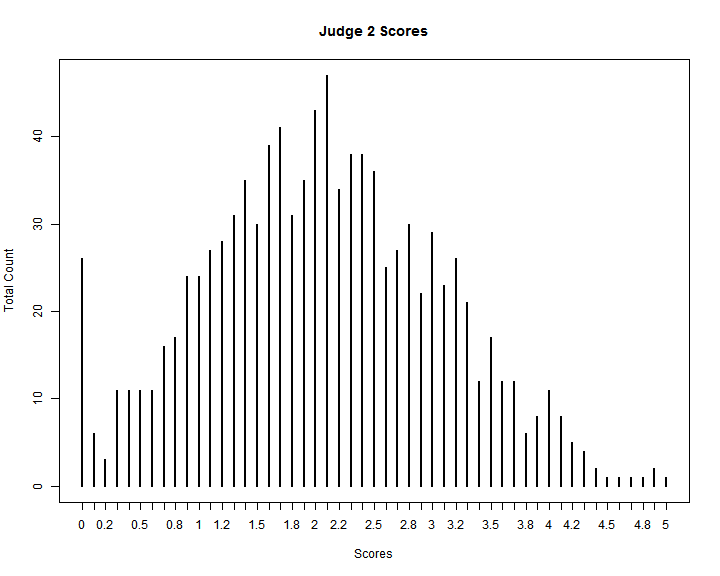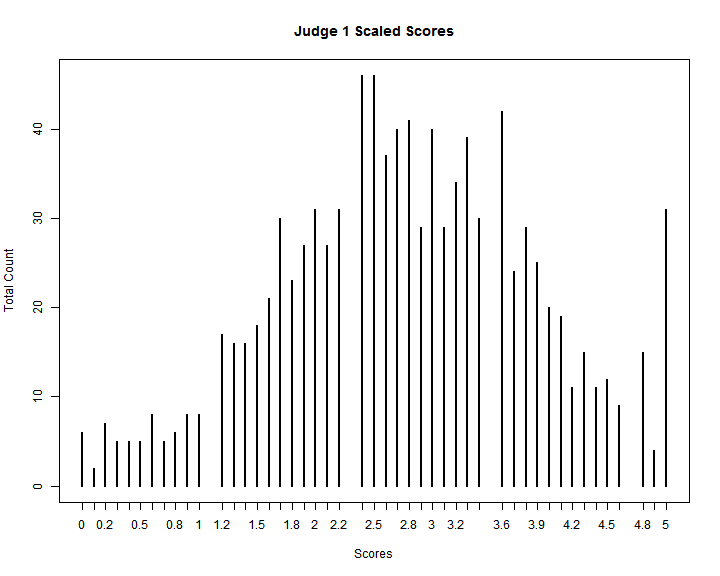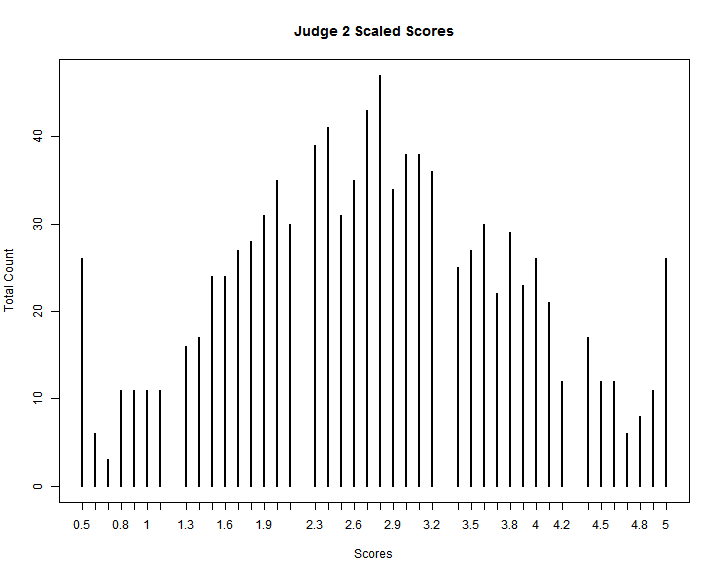The Scoring Algorithm

Once you have completed your application, a minimum of five judges will be assigned to score your proposed project. Judges will offer both scores and comments for each of four distinct traits. Each of the four traits will be scored on a 0-5 point scale, in increments of 0.1. Those scores will combine to produce your total score. Examples of possible scores for a trait are… 0.4, 3.7, 5.0, etc.

The most straightforward way to ensure that everyone is treated by the same set of standards would be to have the same judges score every application; unfortunately, due to the number of applications that we will receive, that is not possible — it would take too long and require too much of each person.

Since the same judges will not score every application, the question of fairness needs to be carefully explained. One judge scoring an application may be very harsh and give everyone a 1.0, while another judge may be more lenient and give everyone a 5.0. So, how do we make sure that no one is penalized (or given an unfair advantage) because of the judges that they are assigned?

Let’s look at the scores from two hypothetical judges:The first judge is a lot less strict than the second judge, who gives much lower scores. If your application was rated by the first judge, it would have a much higher total score than if it was assigned to the second judge.

We have a way to address this problem. We make sure that no matter which judges are assigned to you, your application will be treated fairly. To do this, we utilize a mathematical technique relying on two measures of distribution, the mean and the standard deviation.

The mean takes all the scores assigned by a judge, adds them up, and divides them by the number of scores assigned, giving us an average score. So, if a judge is lenient, he will have a much higher average score than a harsh judge.

Formally, we denote the mean like this:

$\overline{x} = \frac{1}{n} \sum_{i=1}^{n} x_{i}$

The standard deviation measures the “spread” of a judge’s scores. So, maybe two judges both give the same mean (average) score, but one gives a lot of zeros and fives, while the other gives a lot of ones and fours. It wouldn't be fair to you if we didn’t consider this difference.

Formally, we denote the standard deviation like this:

$\sigma = \sqrt{\frac{\sum_{i=1}^{n} (X_{i}-\overline{X})^2}{n-1}}$

So, to ensure that the judging process is fair, we rescale all the scores to match the judging population. In order to do this, we measure the mean and the standard deviation of all scores across all judges. Then, we change the mean score and the standard deviation of each judge to match.

We rescale the standard deviation like this:

$x_{i} = \frac{x_{i}}{(\sigma_{judge}/\sigma)}$

Then, we rescale mean like this:

$x_{i} = x_{i}-(\overline{x}_{judge}-\overline{x})$

Basically, we are finding the difference between both the distributions for a single judge and those for all of the judges combined, then adjusting each score so that no one is treated unfairly according to which judges they are assigned. If we apply this rescaling process to the same two judges in the example above, we can see the outcome of the final resolved scores; they appear more similar, because they are now aligned with typical distributions across the total judging population.